## Direct Search Factorization

Direct search factorization is the simplest Prime Factorization Algorithm. It consists of searching for factors of a number by systematically performing Trial Divisions, usually using a sequence of increasing numbers. Multiples of small Primes are commonly excluded to reduce the number of trial Divisors, but just including them is sometimes faster than the time required to exclude them. This approach is very inefficient, and can be used only with fairly small numbers.

When using this method on a number, only Divisors up to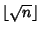(whereis the Floor Function) need to be tested. This is true since if all Integers less than this had been tried, then(1)

In other words, all possible Factors have had their Cofactors already tested. It is also true that, when the smallest Prime Factor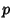ofis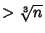, then its Cofactor(such that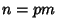) must be Prime. To prove this, suppose that the smallestis. If, then the smallest valueandcould assume is. But then(2)

which cannot be true. Therefore,must be Prime, so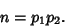(3)